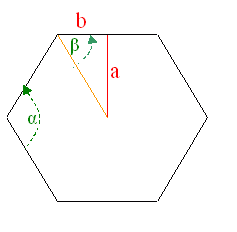Subject: AREA OF A HEXAGON please answer: find the area of a regular hexagon with a apothem of 9 I am a student in the 11 grade Hi there. Take a look at this earlier problem from Esther which is almost identical to yours. One thing you'll need to do first is to determine the length of the sides of the hexagon. Let's label some things on this diagram:You should be able to figure out what the angle α is. From that, you should also see that angle β is just half of that. That gives you a right triangle for which you know a (the apothem) and an angle, so you can use the tangent function to find the length b which represents the other leg of that triangle. With the legs of the right triangle, you can find its area the usual way and then multiply by the number of triangles in the hexagon. Regards, Stephen La Rocque.